# JP2013543365A - Self-sensing of dielectric elastomers using planar approximation - Google Patents

## Info

Publication number
JP2013543365A
JP2013543365A JP2013534847A JP2013534847A JP2013543365A JP 2013543365 A JP2013543365 A JP 2013543365A JP 2013534847 A JP2013534847 A JP 2013534847A JP 2013534847 A JP2013534847 A JP 2013534847A JP 2013543365 A JP2013543365 A JP 2013543365A
Authority
JP
Japan
Prior art keywords
de
equation
dea
method
capacitance
Prior art date
Legal status (The legal status is an assumption and is not a legal conclusion. Google has not performed a legal analysis and makes no representation as to the accuracy of the status listed.)
Granted
Application number
JP2013534847A
Other languages
Japanese (ja)
Other versions
JP6207395B2 (en
JP2013543365A5 (en
Inventor
アラン ギスビー，トッド
マーク オブライエン，ベンジャミン
アレクサンダー アンダーソン，イアン
Original Assignee
オークランド ユニサービシズ リミテッドＡｕｃｋｌａｎｄ Ｕｎｉｓｅｒｖｉｃｅｓ Ｌｉｍｉｔｅｄ
ギスビー，トッド アランＧＩＳＢＹ， Ｔｏｄｄ Ａｌａｎ
オブライエン，ベンジャミン マークＯ‘ＢＲＩＥＮ， Ｂｅｎｊａｍｉｎ Ｍａｒｃ
アンダーソン，イアン アレクサンダーＡＮＤＥＲＳＯＮ， Ｉａｉｎ Ａｌｅｘａｎｄｅｒ
Priority date (The priority date is an assumption and is not a legal conclusion. Google has not performed a legal analysis and makes no representation as to the accuracy of the date listed.)
Filing date
Publication date
Priority to NZ58864110A priority Critical patent/NZ588641A/en
Priority to NZ588641 priority
Priority to NZ59213111 priority
Priority to NZ592131 priority
Application filed by オークランド ユニサービシズ リミテッドＡｕｃｋｌａｎｄ Ｕｎｉｓｅｒｖｉｃｅｓ Ｌｉｍｉｔｅｄ, ギスビー，トッド アランＧＩＳＢＹ， Ｔｏｄｄ Ａｌａｎ, オブライエン，ベンジャミン マークＯ‘ＢＲＩＥＮ， Ｂｅｎｊａｍｉｎ Ｍａｒｃ, アンダーソン，イアン アレクサンダーＡＮＤＥＲＳＯＮ， Ｉａｉｎ Ａｌｅｘａｎｄｅｒ filed Critical オークランド ユニサービシズ リミテッドＡｕｃｋｌａｎｄ Ｕｎｉｓｅｒｖｉｃｅｓ Ｌｉｍｉｔｅｄ
Priority to PCT/NZ2011/000214 priority patent/WO2012053906A1/en
Publication of JP2013543365A publication Critical patent/JP2013543365A/en
Publication of JP2013543365A5 publication Critical patent/JP2013543365A5/ja
Application granted granted Critical
Publication of JP6207395B2 publication Critical patent/JP6207395B2/en
Application status is Active legal-status Critical
Anticipated expiration legal-status Critical

## Images

•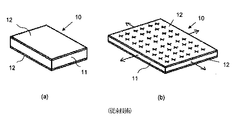••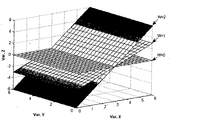•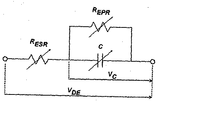•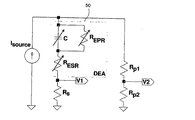•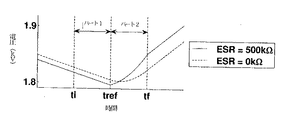•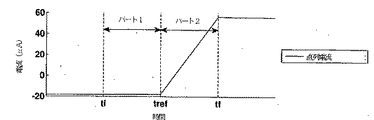•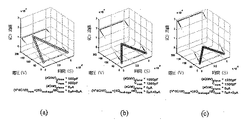••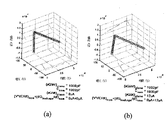•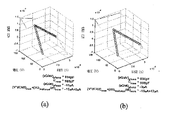•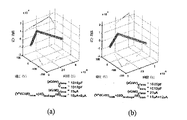•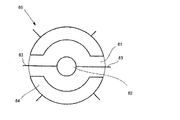••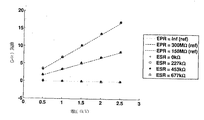•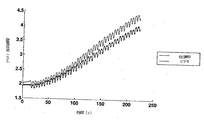•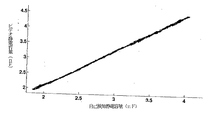•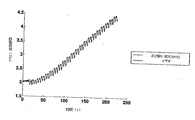•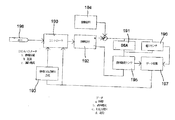## Classifications

• GPHYSICS
• G01MEASURING; TESTING
• G01RMEASURING ELECTRIC VARIABLES; MEASURING MAGNETIC VARIABLES
• G01R27/00Arrangements for measuring resistance, reactance, impedance, or electric characteristics derived therefrom
• G01R27/02Measuring real or complex resistance, reactance, impedance, or other two-pole characteristics derived therefrom, e.g. time constant
• G01R27/26Measuring inductance or capacitance; Measuring quality factor, e.g. by using the resonance method; Measuring loss factor; Measuring dielectric constants ; Measuring impedance or related variables
• G01R27/2617Measuring dielectric properties, e.g. constants
• HELECTRICITY
• H01BASIC ELECTRIC ELEMENTS
• H01LSEMICONDUCTOR DEVICES; ELECTRIC SOLID STATE DEVICES NOT OTHERWISE PROVIDED FOR
• H01L41/00Piezo-electric devices in general; Electrostrictive devices in general; Magnetostrictive devices in general; Processes or apparatus specially adapted for the manufacture or treatment thereof or of parts thereof; Details thereof
• H01L41/02Details
• H01L41/04Details of piezo-electric or electrostrictive devices
• H01L41/042Drive or control circuitry or methods for piezo-electric or electrostrictive devices not otherwise provided for
• HELECTRICITY
• H02GENERATION; CONVERSION OR DISTRIBUTION OF ELECTRIC POWER
• H02NELECTRIC MACHINES NOT OTHERWISE PROVIDED FOR
• H02N2/00Electric machines in general using piezo-electric effect, electrostriction or magnetostriction
• H02N2/02Electric machines in general using piezo-electric effect, electrostriction or magnetostriction producing linear motion, e.g. actuators; Linear positioners ; Linear motors
• H02N2/06Drive circuits; Control arrangements or methods
• H02N2/062Small signal circuits; Means for controlling position or derived quantities, e.g. for removing hysteresis

## Abstract

The present invention provides a method for obtaining feedback parameters relating to the state of a dielectric elastomer (DE). The method introduces small-scale vibrations into the voltage difference between the electrodes of the DE, monitors or repeatedly measures several measurable electrical properties of the DE, and derives other relevant data from the measurements. And, when defined as orthogonal axes, deriving an equation for the best-fit plane via the relevant data and deriving feedback parameters from the coefficients of the plane equation. Thus, the method provides important feedback regarding DE capacitance, leakage current and / or electrode resistance. Also disclosed are computer programs and systems adapted to perform the methods.
[Selection] Figure 19

## Description

The present invention relates to a method and apparatus for sensing electrical parameters of a dielectric elastomer (DE). More particularly, the present invention relates to self-sensing by dynamically estimating the electrical parameters of the DE and deriving an estimate of the state of the DE from those parameters.

Dielectric elastomers (DE) can be used to convert electrical energy into mechanical energy, or vice versa. The DE can be used as an actuator, generator, and / or sensor.

Referring to FIGS. 1 (a) and 1 (b), a DE (generally indicated at 10) comprises a dielectric elastomer film 11 that is typically provided between flexible electrodes 12 and cannot compress the capacitance.

Dielectric elastomer actuators (DEA) have a voltage difference of, for example, “Electrostriction of polymer dielectrics with compliant electrodes as a means of actuation” (Pelline R. E., Kornblh R. D., Konbhl R. D.). As disclosed in Sensors and Actuators, A, Physical 64 (1), pages 77-85 (1998)), it is deformed when applied to both ends of the two electrodes 12. The charge accumulates on the electrode 12 in a capacitor manner, and from the resting or stretching state as shown by the example of FIG. A static voltage is generated that results in the operating or inflated state shown in FIG.

If the planar dimension of the DEA is much larger than its thickness, the pressure magnitude is defined by the following equation: Where P is the pressure, ε r is the dielectric constant of the dielectric material, ε 0 is the free space dielectric constant (8.854 × 10 −12 F / m), V is the voltage, and d is It is the dielectric film thickness.

On the other hand, a dielectric elastomer generator (DEG) converts mechanical energy into electrical energy. In practice, DEG is a variable capacitor, and its capacitance changes with mechanical strain (ie, membrane deformation).

The DEG generates electrical energy by increasing the potential energy stored therein. The steps for achieving this are shown schematically in FIG. Starting from the top of FIG. 2, mechanical energy 20 is applied to DEG 21 by first stretching it. This causes planar expansion of the electrode 22 and orthogonal compression of the membrane 23, increasing the capacitance. The electrical energy 24 is then input to the DEG by charging (or priming) from a power supply (not shown) so that the opposing electrode 22 is reverse charged. By relaxing the DEG, mechanical energy can be obtained by separating the opposite charges (+ and-) on the opposing electrodes 22 and by causing the same charges on each electrode 22 that is close to each other due to its reduced plane. Is converted into electrical energy. Electrical energy 24 is extracted and the cycle is repeated.

Dielectric elastomers may alternatively or additionally be used as sensors. The electrical properties of the dielectric elastomer sensor, such as capacitance, electrode resistance, and equivalent parallel resistance of the dielectric film, change in response to external stimuli, such as mechanical deformation or changes in the DE operating environment. Thus, feedback information can be collected using changes in the electrical parameters of the DE.

For many practical applications of DEA, DEG, and DE sensors, it is generally necessary or at least preferred to obtain feedback on the instantaneous state of the DEA for sensing and control purposes. For DEA and DEG, coupling them directly to individual rigid strain, displacement, velocity or acceleration sensors inevitably increases the cost and mass of the device, and such strategies are kinematically suppressed. It is effective for a conventional rigid device that is a part of the structure, while suppressing the movement of DEA / DEG unnecessarily. Similarly, attaching the sensor to the rigid body to which the DE is connected may provide useful feedback regarding the machine output, but provides limited information regarding the state of the electrical machine of the DE itself. Therefore, it is important to use DE itself as a sensor and to combine detection with actuation and / or power generation, hereinafter referred to as a “self-sensing” function.

It is possible to correlate changes in DE capacitance with changes in the physical shape of the DE. The self-sensing effect in the prior art has focused on combining sensing with actuation. “An adaptive control method for selective elastomer devices” (Todd A. Gisby, Emilio P. Calius, Shane Xie, DE from Ie A. Anderson, Ai Ason To provide feedback, the use of self-sensing based on capacitance between electrodes is disclosed. A similar method is described, for example, in “Control system design for a selective elastomer actuator: the sensory of St. L. A. and A. Goldenberg, Toe, L. A. and A. A. Golden et. analysis of dielectric elastomer actuators "(Keplinger, C., Kaltenbrunner, M., Arnold, N., and Bauer, S., Applied Physics Letters, ns, and es, c). ric elastomer actuator "(. Jung, K., K.J Kim, and H.R. Choi al., Sensors and Actuators A: Physical, 2008) has been disclosed by.

International Patent Publication No. WO2010 / 095960, entitled “System and Method for Dynamic Self-Sensing of Dielectric Elastomer Actuators”, estimates the capacitance difference of DEA, the potential difference between the capacitances of DEA, An improved method and system for self-sensing is disclosed by deriving from the rate and current through the DEA. From the capacitance, an estimate of the charge and physical state of the DEA can be derived. It is also possible to derive an estimate of the leakage current through the dielectric film.

However, this and other self-sensing methods of the prior art usually have one or more disadvantages. These are accurate only under certain circumstances, such as when the DE is stationary (ie not subject to any perturbations caused by external forces), the leakage current is very low, or the operating speed is low. May include certain estimates. Alternatively, or additionally, prior art methods and / or devices that obtain estimates based on other assumptions that may not necessarily be applicable are suitable for practical implementation in systems designed for portable use. Rather, it is sensitive to noise and / or requires unnecessarily complex, bulky or processor intensive calculations to be performed

Providing a method and apparatus for a dielectric elastomer actuator or generator self-sensing or dielectric elastomer sensor that overcomes or at least ameliorates one or more disadvantages of the prior art, or alternatively, a generally beneficial choice It is an object of the present invention to provide at least.

Further objects of the present invention will become clear from the following description.

In one aspect, the present invention may be said to be a method for estimating the state of a dielectric elastomer (DE) in a broad sense, the method comprising:
Introducing a small-scale vibration into the voltage difference between the electrodes of the DE;
Measuring the potential difference across DE and the series current through DE over a period of time;
Deriving an equation for the best-fit plane through data derived from said measurements when defined as orthogonal axes;
Deriving one or more feedback parameters from the coefficients of the plane equation.

Preferably, the feedback parameter includes the capacitance of DE, which is approximated by a first coefficient of the plane equation.

Preferably, the first coefficient is a voltage variable coefficient of a plane equation.

Preferably, the feedback parameter further comprises a leakage current through the DE, the leakage current being derived from the second coefficient of the plane equation.

Preferably, the second coefficient is a coefficient of a time variable of the plane equation.

Preferably, the period during which the potential difference across DE and the series current through DE is measured is short compared to the mechanical and electrical time constants of the DE system.

Preferably, the period is equal to or longer than the period of small-scale vibration.

Preferably, deriving an equation for the best fit plane includes performing a regression analysis of the data derived from the measurements.

Preferably, deriving the equation for the best fit plane includes solving a set of linear equations.

Preferably, the data derived from the measurements includes the potential difference across DE, the series current through DE, the charge supplied to DE, and the time, and the best fit plane includes the hyperplane.

Preferably, the feedback parameter further comprises an equivalent series resistance of DE, which is derived from the third coefficient of the hyperplane equation.

Preferably, the third coefficient is a current variable coefficient of the hyperplane equation.

Preferably, the equivalent series resistance is approximated by dividing the third coefficient of the hyperplane equation by the capacitance of DE.

Preferably, the leakage current is derived from the second factor of the plane equation by subtracting the nominal synergistic effect of the change rate of the DE capacitance and the change rate of the equivalent series resistance of the DE from the second factor.

Preferably, the leakage current is derived using the capacitance time history and the DE equivalent series resistance.

Preferably, the DE leakage current approximation is derived using the capacitance and equivalent series resistance approximation obtained according to the method of the present invention.

Preferably, the first coefficient of the plane equation corresponds to the partial derivative of the charge supplied to the DE with respect to the potential difference across the DE.

Preferably, the second coefficient corresponds to the partial derivative of the charge supplied to the DE with respect to time.

Preferably, the third coefficient corresponds to the partial derivative of the charge supplied to the DE with respect to the series current through the DE.

Preferably, the data derived from the measurements includes the potential difference across the DE capacitive component, the charge supplied to the DE, and the time.

Preferably, the time, the potential difference across the DE capacitive component, and the charge supplied to the DE are defined as orthogonal x-axis, y-axis, and z-axis, respectively.

Preferably, the leakage current is derived from the second factor of the plane equation by subtracting from the second factor the product of the rate of change of the DE capacitance and the potential difference across the DE capacitive component. .

Preferably, the rate of change of the capacitance of the DE is derived using the time history of the capacitance obtained according to the method of the present invention.

Preferably, the method comprises
Supplying a pulse width modulation (PWM) periodic actuation signal to the DE;
Measuring the step change in voltage across DE, and the step change in current through DE in the transition of the PWM actuation signal;
Further comprising estimating the DE equivalent series resistance (ESR) by dividing the step change in voltage across the DE by the step change in current through the DE.

Preferably, estimating the ESR of DE is
Recording an estimated ESR time history;
Deriving an approximation of the ESR with respect to time from the recorded time history of the estimated ESR so that the approximation can be used to estimate the ESR during the transition of the PWM actuation signal And further including.

Preferably, the potential difference across DE is measured by determining the voltage difference between the positive and negative electrodes of DE.

Preferably, the charge delivered to the DE is derived by integrating the series current through the DE with respect to time.

Preferably, the method further comprises associating the capacitance of the DE with the physical state of the DE. This may include, for example, one or more estimates of the planar area, thickness, and / or dimensions of the DE.

Preferably, the method further includes the steps of monitoring leakage current and controlling inflow to the DE system to limit leakage current.

Preferably, the method further comprises the step of associating the equivalent series resistance of DE with the strain of DE.

Preferably, the method further includes controlling the operation of the DEA based on the feedback parameter, thereby forming a closed loop feedback control system.

In a further aspect, the present invention may be referred to in a broad sense as a method for controlling a dielectric elastomer (DE), the method comprising:
Receiving an input signal indicating a required state of the DE;
Obtaining an estimate of the state of the DE according to any one of the foregoing descriptions;
Adjusting one or more system inputs responsive to both the input signal and the estimate so that the estimated state substantially matches the required state.

In a further aspect, the present invention may be said in a broad sense to be a system adapted to perform any one of the foregoing descriptions.

In yet a further aspect, the present invention broadly
A dielectric elastomer (DE) comprising a pair of electrodes separated by an elastomeric membrane;
Power supply means adapted to supply a small voltage oscillation to the DE;
Measuring means for measuring the potential difference across the DE and the series current through the DE;
Computation means communicatively coupled to the measurement means, the computation means for deriving an equation for a plane that best fits the data, such as deriving from measurement data relating to the electrical properties of the DE over a predetermined period of time. And computing means adapted to derive one or more feedback parameters from the coefficients of the plane equation.

Preferably, the measuring means has a sampling frequency higher than the frequency of the predetermined period.

Preferably, the computing means receives one or more system inputs to receive an input signal indicative of the required state of the DE and to provide a closed loop feedback control of the DE based on the feedback parameters. Further adapted to control.

Preferably, the computing means is adapted to perform a regression analysis on the stored data, thereby deriving an equation for the hyperplane that best fits the stored data when defined as orthogonal axes. .

Preferably, the feedback parameter includes one or more of DE capacitance, equivalent series resistance and / or leakage current.

Preferably, the computing means is further adapted to record the feedback parameter.

Further aspects of the invention that should be considered in all of its novel aspects will become apparent from the following description.

A number of embodiments of the invention will now be described by way of example with reference to the drawings.

FIG. 2 shows a prior art dielectric elastomer (DE) showing a resting state and (b) an expanded state. FIG. 3 is a process diagram illustrating the generation of electricity using a dielectric elastomer generator according to the prior art. It is a three-dimensional view of a hyperplane in a four-dimensional space. It is a figure which shows the concentrated circuit model of electricity of DE. FIG. 2 is a schematic diagram of a DEA as part of a simple self-sensing circuit according to the present invention. It is a figure which shows the effect of ESR based on the voltage ( VDEA ) of both ends of DEA . FIG. 4 is a diagram showing series current (i s ) through DEA. FIG. 6 is a diagram illustrating the effect of a capacitance on a matched plane derived according to the second embodiment of the present invention by simulated capacitances of (a) 500 pF, (b) 1000 pF, and (c) 1500 pF. is there. Non-conformal planes derived according to the second embodiment of the present invention with dC / dt values of (a) -500% / s, (b) 0% / s, and (c) 500% / s. It is a figure which shows the influence of zero dC / dt. (A) zero leakage current (ie, infinite EPR), and (b) 250 MΩ equivalent parallel resistance (EPR), in accordance with the second embodiment of the present invention It is a figure which shows an influence. In combination with 250 MΩ EPR in parallel with DEA capacitance, (a) negative dC / dt and zero leakage current, and (b) same negative dC / dt, FIG. 6 shows the synergistic effect of leakage current and negative value for dC / dt on the fitted plane, derived according to the second embodiment. In combination with 250 MΩ EPR in parallel with the DEA capacitance, (a) positive dC / dt and zero leakage current, and (b) same positive dC / dt, FIG. 6 shows the synergistic effect of leakage current and positive value for dC / dt on the fitted plane, derived according to the second embodiment. FIG. 3 schematically shows a magnified dot DEA used to experimentally validate a second embodiment of the method of the invention. FIG. 4 is a graph of estimated and actual ESR versus a range of equivalent series resistance (ESR) and EPR values, the estimated values being derived according to a second embodiment of the present invention. FIG. 6 is a graph of leakage current voltage estimated using the second embodiment of the present invention for a range of ESR and EPR values. FIG. 6 shows a comparison of the capacitance of a test DEA estimated by a second exemplary embodiment of the self-sensing method of the present invention with respect to capacitance measured by a video extensometer. FIG. 6 shows the capacitance of a DEA estimated by a second embodiment of the plotted self-sensing method versus the capacitance estimated by a video extensometer. Second embodiment of a method of self-sensing plotted against capacitance measured by a video extensometer after the estimated capacitance of the DEA has been expanded according to the relationship shown in FIG. It is a figure which shows the electrostatic capacitance of DEA estimated by (1). 1 is a schematic block diagram of an exemplary system for implementing the self-sensing method of the present invention.

Capacitance, leakage current through the dielectric film, and electrode resistance are each relative to the dielectric elastomer (DE), whether used as an actuator (DEA), generator (DEG), or sensor. Is a potentially important feedback parameter.

Capacitance can be related to the instantaneous area of the electrode and the thickness of the dielectric film. It is also possible to estimate the electric field in the dielectric to be evaluated, which is essential for measuring the charge stored on the DE at any point in time. Leakage current is a measure of the overall efficiency of the DE and can be used as part of a condition monitoring system. The resistance of the electrode (equivalent series resistance, ESR) changes as the DE deforms, so the electrode acts in a manner similar to a strain gauge.

Thus, recognition of one or more of the above feedback parameters is desirable for determining or estimating the state of the DEA. A “state” of DEA may refer to, for example, the size, shape, area, thickness, dimension, deformation, electric field, charge, effect, and / or state of DEA.

Each of the above feedback parameters (capacitance, leakage current, and equivalent series resistance) is directly or indirectly affected by the others and thus dynamically as DE is subjected to electrical and mechanical stimuli. Change. It is not uncommon for the relationship between strain and resistance to change over time and with the number of strain cycles, for example. Unfortunately, it is not easy to directly measure the parameter of interest.

According to the prior art, self-sensing has usually been limited to estimating the capacitance of the DEA and the equivalent series resistance (ESR) of the electrodes. This self-sensing has generally been achieved by superimposing a high-frequency, low-vibration AC signal on the actuation signal. The gain of the high frequency signal is proportional to the capacitance once it passes through the DEA and relates the phase delay to the ESR. However, low power DC-DC converters used to generate actuation signals for DEAs for portable devices are usually difficult to modify the output and regenerate high frequency signals. In addition, such an approach prevents power supplies from being used to independently control multiple DEAs, thus each degree of freedom will require its own power supply. A dedicated power source is an inefficient use of capacity and weight when operation is intermittent or when normal operation does not require all of the degrees of freedom to be operated simultaneously.

The present invention provides a method and apparatus that allows the estimation of any or all of these important feedback parameters (capacitance, leakage current, and ESR) in substantially real-time, and thereby their behavior. Reduce the need to make speculations about, improve the accuracy of DE state feedback for improved control and / or monitor over a wide range of conditions.

First Exemplary Embodiment In a first embodiment of the present invention described below by way of example, the method of the present invention provides data on four variables including three electrical properties and time of a dielectric elastomer (DE). Involved in the derivation of These four variables are defined as orthogonal axes that form an abstract four-dimensional space, so the best-fit plane through the data includes the three-dimensional hyperplane. The DE feedback parameter can be derived from the coefficients of the equation for the hyperplane.

The hyperplane is an (n−1) -dimensional subspace of the n-dimensional space. This can be described in terms of an n-dimensional space coordinate system by a single linear equation. For example, in a three-dimensional space, a two-dimensional space with a characteristic equation of the form z = ax + by + c is a hyperplane (where x, y, and z are orthogonal axes in the three-dimensional space and a, b And c are constants). Throughout this specification, the term “hyperplane” when used in describing preferred embodiments of the present invention refers to a three-dimensional subspace in a four-dimensional space. The “hyperplane” characteristic equation is of the form z = ax + by + cw + d, where x, y, z, and w are orthogonal axes in four-dimensional space, and a, b, c, and d are constants Is).

Although a three-dimensional hyperplane in four-dimensional space cannot be easily illustrated, FIG. 3 provides a three-dimensional representation of the hyperplane in four-dimensional space to illustrate the concept. The X, Y, and Z dimensions of the hyperplane were shown as orthogonal axes on the 3D diagram, and the hyperplane was discretized in the fourth dimension (W). This can be done, for example, using a two-dimensional contour plot where X and Z are shown on the orthogonal axes, and individual lines on the contour plot represent discrete values of Y (X, Y, And Z) in two dimensions.

A DE electrical lumped circuit model is shown in FIG. DE can be represented as a variable capacitor (C) in parallel with a variable resistor (representing the equivalent parallel resistance of DE, R EPR ) or can be modeled, both in series with another variable resistor Connected (represents the equivalent series resistance of DE, R ESR ).

The electrical characteristics of the DE that can be easily measured are the voltage V DE across the DE as a whole (ie, not the potential difference between the electrodes 12, but represented by the capacitor C between the terminals of the DE) and the series through the DE. Current (i S ).

The voltage across the capacitor (V C ) subtracts the product of the series current (i S ) through DE and the ESR of the electrode (R ESR ) from the voltage across V DE (V DE ), as shown by Equation 1. .

The current through the DE (i S ) is equal to the sum of the current through the capacitive element (i C ) and the leakage current through the EPR (i EPR ) (Equation 2).

The present invention repeatedly measures and derives data from certain electrical properties of the DE, derives equations for the best-fit hyperplane through the data, and derives DE feedback parameters from hyperplane coefficients To derive estimates of capacitance (C), leakage current (in the DE model, current through equivalent series resistance R ESR , i EPR ) and equivalent series resistance ESR (R ESR ).

When using a small, high frequency vibration signal to introduce vibration into the pressure across the DE, a feedback data window spanning a period greater than or equal to the period of the vibration component of the signal is used to set the DE feedback parameter. Can be determined. The present invention is not limited to any particular waveform for the vibration signal. Suitable waveforms include, but are not limited to, for example, square waves, sine waves, triangular waves, or sawtooth waves.

The first step of the method is to obtain and record a measurement of the potential difference or the voltage across DE (V DE ) and the series current (i S ) through DE during a short time window. The time window should be short compared to the mechanical and electrical time constants of DE. Conveniently, the window length is set to correspond to the period of small oscillations superimposed on the voltage across DE.

The measurement of the DE voltage V DE and the series current i S is made at various times within the period. Also, from the latter measurement, the charge input (Q input ) to the DE over time can also be derived (ie, as an integral of the series current i S over time) and recorded.

The measurements are preferably made at a sampling rate substantially higher than the frequency of the time window, giving a plurality of data points during each time window.

Short compared to the electrical and mechanical time constant of DE, charges input to the DE over a period (Q input The) is, t = 0 and t = time of the current through the capacitive element of DE between t a and, time t = 0 and t = t a integral (charge Q c (0) is equivalent to minus the in the charge Q c (t a) from t = 0 on the capacitor in which t = ta) regarding equal to the sum of the integral Q leakage (t a) with respect to time of the leakage current i EPR between can show (equation 3).

Because of the short duration, it can be assumed that C can be modeled using a first order approximation, i.e. dC / dt is substantially constant. If the maximum amplitude of V c for the window period is sufficiently small, it can be assumed that the leakage current (i EPR equivalent to dQ leakage / dt) is also substantially constant. Applying these assumptions to Equation 3 and expressing the charge with respect to C and V c yields Equation 4.

Section V c in the first term of right hand side of Equation 4 may be replaced by an equivalent term including ESR dependent terms, i.e., V c becomes V DE -i s R ESR, equations 5 Brought about.

Thus, instead of obtaining the voltage across the DE capacitive component (V c ), the voltage across the DE itself (V DE ) can be used. Unlike V c , V DE is an electrical characteristic of a circuit that can be easily measured.

Equation 5 representing the relationship between the electrical parameters of the DEA at a t = t a.

If t, V DE , i s , and Q input are defined as orthogonal axes in 4D space, the plurality of recorded data points are all substantially cubic when plotted against those axes. It was found to fall on the original hyperplane or adjacent to the three-dimensional hyperplane. A large number of data points are preferably recorded to ensure that the planar fit is well adjusted. However, a relatively small number of data points (at least 4 or more) may be acceptable in certain applications. The appropriate number of data points (and thus the sampling rate of measurement) for a particular fit will be apparent to those skilled in the art.

An important part of successfully fitting the hyperplane to available data points is the vibration introduced into the voltage across DE. Without charge / discharge oscillations, Q input , V c , and t data will fall into lines or 2D planes in 4D space. The system will be underdetermined and therefore it will be impossible to find its own hyperplane that passes through all data points. This prevents the process of matching the plane from functioning and prevents accurate determination of the electrical characteristics of the DE.

Hyperplane coefficients can be estimated and associated with all three major feedback parameters of the DE. For example, regression can be used to find the hyperplane coefficients that best represent the recorded data points (ie, the best-fit hyperplane) for t, V DE , i s , and Q input . However, other methods of estimating hyperplane coefficients may alternatively be used without departing from the scope of the present invention. The term “best-fit hyperplane”, as used herein, each corresponds substantially to the “gradient” of data points along its same axis, in whatever way the plane may be derived. Only a hyperplane in which at least most of the data points in the 4D plot of potential difference, current, charge, and time are provided on or substantially adjacent to it, provided with a slope or gradient of the plane along the axis. Absent.

The hyperplane coefficient formed by the recorded data points is equal to the partial derivative of Q input (Equation 5) with respect to t, i s , and V DE . That is, the coefficients associated with each of the hyperplane equation variables (t, V DE , i s , and Q input ) represent the slope of the hyperplane along the respective axis. Given that each of the data points falls substantially on the hyperplane, these gradients are equal to the aforementioned partial derivative of Equation 5, so the DE feedback parameter is Can be derived from the coefficients.

Referring to Equation 5, dR ESR / dt can be assumed to be a constant, as well as dC / dt and dQ leakage / dt over a short period of time or an associated measurement window. The term C (0) V c (0) is a constant and therefore does not appear in the partial derivative.

The Q input partial derivative with respect to V DE (equal to the first coefficient of the hyperplane equation) is the nominal capacitance of DE over the period of the feedback window (C in Equation 6, C of time for clarity). omit subscripts), the partial derivative of the Q input the related i s a (equal to what is called the third coefficient of hyperplane equation) dividing in C, and become a nominal ESR of the electrode to the period of the feedback window (equation R ESR of 7 (time subscripts omitted for clarity), Q input partial derivative with respect to t (equal to what is called the second coefficient of the hyperplane equation) is the rate of change in capacitance , The rate of change of the ESR of the electrode, and the nominal synergy of leakage current (Equation 8, time subscripts omitted for clarity).

Thus, an approximation or estimate of the DE capacitance C can be derived directly from the slope of the best-fit hyperplane along the voltage axis V DE (relative to the Q input axis), ie the voltage variable coefficient in the equation is the best A conforming hyperplane is defined.

Similarly, approximate the equivalent series resistance R ESR of DE derives by dividing the electrostatic capacitance C of the approximation before the gradient of the hyperplane along (with respect to Q input The axis) series current i s axis be able to.

Using the time history of C and R ESR calculated using Equations 6 and 7, respectively, dC / dt and dR ESR / dt (both assumed to be substantially constant) can be calculated. , DC / dt and dR ESR / dt can be used to identify the individual terms of Equation 8, and in particular the leakage current i EPR equal to the final term dQ leakage / dt.

This embodiment of the invention has several advantages over the second embodiment described below and / or the prior art, and includes one or more of the following.
1. The process of fitting the hyperplane is accomplished using a single stage mathematical process.
2. There are few stringent requirements on the waveform used to generate small-scale oscillations in the voltage across DE. That is, the method does not necessarily require a PWM power supply, thereby increasing flexibility with respect to hardware design.
3. Capacitance is obtained directly by fitting the hyperplane to available data.
4). The process is insensitive to noise for the following reasons.
a. The series current integration (to obtain Q input ) acts as a means for low pass filtering the series current feedback data. If the noise has an average value of zero, the integration acts to attenuate the noise component from the signal.
b. To use regression to fit a plane to available data, use all available data. This is not due to data points from any one point in time, and therefore the influence of pseudo data points on the estimated parameters is minimized.

It also provides a mechanism for evaluating the validity of the constants dR ESR / dt, dC / dt, and dQ leakage / dt assumptions through the use of least squares regression. If the assumption is valid, the rest of the regression process is small. However, a large residual indicates that one or more assumptions are not met.

Second Exemplary Embodiment As a further illustration of the method of the present invention, according to a second embodiment described below, the method comprises three electrical properties and time of dielectric elastomer (DE), including three Involved in deriving data about variables. These three variables are defined as the orthogonal x-axis, y-axis, and z-axis that form a three-dimensional space, so the best-fit plane through the data includes the plane.

This second illustration of the present invention will now be described with respect to application of a method associated with a dielectric elastomer actuator (DEA) system. However, the method may be applied to dielectric elastomer generators or sensors as well.

The DEA system uses pulse width modulation (PWM) control of input power (actuation signal) to simultaneously operate the DEA and introduce small-scale vibrations into the voltage difference between the DE electrodes. This enables the DEA capacitance, leakage current through the equivalent parallel resistance (EPR) of the dielectric film, and dynamically estimated equivalent series resistance (ESR) of the electrode, while the DEA is activated. . PWM can easily be replaced with a digital computer and can be used to power a plurality of independent DEAs simultaneously with a single power supply installed at a fixed voltage.

By making the duration of the PWM signal sufficiently small compared to the mechanical and electrical time constant of the DEA, the control of the signal duty cycle controls the average voltage seen across the DEA, which Manage the degree. At the same time, rapid switching of the PWM signal introduces small-scale oscillations into this voltage. Thus, this method clearly demonstrates a dynamic change in electrode resistance, a change to the conductivity of the dielectric film at high electric fields, and a change in capacitance of the DEA as the DEA is activated and / or relaxed. It becomes a factor of the current induced in the rate.

Although the method and apparatus / system of the second exemplary embodiment has been disclosed to include a PWM power supply, PWM is not an essential feature of the present invention. Any other time-varying signal, such as an amplitude-modulated actuation signal, with an overlaid high frequency vibration signal is alternatively used to allow detection of DEA capacitance and leakage current. Also good. It should be understood that the planar fitting method of the present invention may be used to extend many existing or future methods of self-sensing, such as at least the method disclosed by Keplinger et al. However, the PWM power supply provides a number of advantages in this second exemplary embodiment of the present invention.

As previously described in the first exemplary embodiment, it is necessary to measure the series current i s through the DEA and the voltage or potential difference V DEA between the positive and negative terminals of the DEA . Referring again to FIG. 4, the DEA can be modeled as a variable capacitor (C) connected in parallel with a variable resistor (R EPR ), both of which are in series with another variable resistor (R ESR ). Connected.

A schematic of the DEA system is shown in FIG. 5 and has a DEA 50 represented by the components of the model of FIG. 4 inside a dashed square.

The power supply of the DEA system is preferably a high voltage DC-DC converter connected to the DEA via a high voltage, high speed optocoupler. The current flowing from the DC-DC converter to the DEA via the PWM switch is proportional to the input current to the optocoupler. Therefore, both the DC-DC converter and the optocoupler behave as a current source (I source ). The PWM signal is used to control the input current to the optocoupler. A 30 kΩ fixed resistor (R s ) is used to measure the series current through the DEA (by measuring voltage V1 and applying Ohm's law), 100 MΩ: 120 kΩ (R p1 and R p2 respectively) ) Resistor ladder (voltage divider) is used to measure the voltage V2 at the positive terminal of the DEA 50 and provide a passive discharge path for the DEA.

Unlike the first example of the present invention described above, this second example method is based on the potential difference (V c ) across the capacitive component of the DEA, the charge supplied to the DEA (Q input ), and measurements. We need data about the derived time (t).

By plotting the charge supplied to the DE (Q input ), the voltage across the capacitive component of the DE (V c ), and the time t on the orthogonal axis, it is fit to the data drawn in 3D space. It is possible to reduce the hyperplane of the first exemplary embodiment to a further 2D plane.

If the ESR is significant, the potential difference across the DEA (V DEA ) is subtracted from the potential difference across the ESR (V ESR ), and as shown by Equation 9, the potential difference across the DEA capacitive component (V c ) Must be determined.

For example, if the power delivered to the DE is controlled using pulse width modulation (PWM), the equivalent series resistance R ESR is estimated at a certain time when the PWM signal transitions between a high state and a low state. can do. As the PWM signal transitions, the step change in series current through DE results in a step change in voltage across DE. Since the potential difference V c across the DE capacitive component cannot be changed immediately, R ESR is the magnitude of the step change in the voltage V DE across the DE, the series current through the DE through the PWM signal transition. equal divided by the size of the step change in i s. By recording the time history of the calculated resistance value, the n-th order approximation of RESR over time can be used to estimate the electrode resistance at a fixed time during the PWM transition.

Other methods of detecting DEA ESR may alternatively be used without departing from the scope of the present invention. For example, if PWM input signal slewing is limited, ESR can be detected by considering two short periods (parts 1 and 2 of FIGS. 6 and 7) for PWM signal transitions. is there. Part 1 begins just before the start of the PWM input signal cycle and ends at the start of the PWM cycle. Part 2 continues from the end of Part 1 and begins at the beginning of the PWM cycle and ends once the PWM input signal reaches its peak value. At the beginning of part 1 is t i, at the end of part 1 / at the start of part 2 is t ref , and at the end of part 2 is t f . When the ESR is negligible, V DEA is approximately equal to V c and V DEA shows a smooth transfer from the discharge to the charged phase during Part 2, as shown in FIG. 6 (dashed line). . Although otherwise constant, again as shown in FIG. 6 (solid line), significant ESR introduces a discontinuous or sharp angle into the voltage waveform at t = t ref . This discontinuity becomes more pronounced, i.e., the corners become sharper as the ESR increases.

By assuming that ESR is constant for t 1 <t <t 2 , ESR can be estimated by transforming V DEA to remove discontinuities. This requires the execution of a four curve fitting operation. By shortening the duration of the PWM signal relative to the electrical time constant of the system, the series current (i s ) through the DEA and the potential difference across the DEA (V DEA ) during part 1 and between part 2 The series current (i s ) through the DEA is approximately linear (Equations 10-12), as shown in FIGS. Assuming that both R EPR and the time derivative of capacitance are substantially constant at a particular point in time (linearization point), it is limited by applying the lamp input current (ie, at the PWM input). Slew rate), resulting in V DEA drawing a parabola along the time dimension. Therefore, a second order fit is required for V DEA during Part 2 (Equation 13).

Substituting adapted curve equation 9 (resulting in equations 14 and 15), the derivative with respect to time of V c for Part 1, in order to remove the discontinuity, V c at t = t ref against Part 2 Must be equal to the derivative of time. Thus, by equalizing the first derivative with respect to time of V c at t = t ref for parts 1 and 2, the equation for R ESR shown in equation 16 can be derived.

The limited slew rate on the PWM signal provides a smooth transition from discharge to charge and vice versa. This removes the switching noise, ensures that V c is a continuous function over time, and allows R ESR to be estimated. The coefficients of Equation 13 that fit the raw data in least squares detection can be found by solving simultaneous equations, which can be achieved in a straightforward manner using, for example, the Kramer formula. This process is also substantially convenient because many of the required sums can be pre-computed, and those that cannot be updated can be updated as each data point becomes available.

Once the equivalent series resistance is known or estimated, the capacitance of the DEA can be converted to this second exemplary implementation of the present invention by any of the above methods, or any other alternative means. It can be calculated according to the method of form.

Referring to the branch circuit in FIG. 5 representing the DEA 50, the voltage across the capacitor (V c ) is derived from the voltage across the DEA 50 (V DEA ) as shown by Equation 17 (derived from Equation 9). Reduce the product of the series current through the DEA (i s ) and the electrode ESR (R ESR ).

The series current (i s ) through the DEA branch circuit is equal to the sum of the current (i c ) through the capacitive element and the leakage current (i EPR ) through the EPR (Equation 18).

When a small, high frequency vibration signal is superimposed on the actuation signal supplied to the DEA, a feedback data window spanning one or more periods of the vibration component of the signal is used to static the DEA. Capacitance (C) and leakage current (i EPR ) can be determined. PWM has been used to generate the vibration component of the actuation signal, but if Vc is known, other methods for generating periodic vibration can be used without departing from the scope of the present invention. , C and leakage current can be determined.

It is advantageous to set the width of the feedback data window to match the period of the PWM signal. We define time (t) and the charge stored on DEA (Q c ) to be zero at the beginning of the window. As in the first exemplary, input charge Q input The (t a) to DEA at time t a is defined by Equation 3 and 4.

The coefficients of the 2D plane formed by the t, V c , and Q input data are equal to the partial derivatives of Q input in Equation 4 with respect to t and V c . That is, the partial derivative (first coefficient) of Q input with respect to V c is the capacitance (C) (Equation 19, time subscript omitted for clarity). The partial derivative (second coefficient) with respect to the time of Q input is a synergistic effect of the rate of change of capacitance (V c ∂C / ∂t) and the leakage current through the dielectric film (∂Q leakage / ∂t). Yes (Equation 20, time subscript omitted for clarity).

Thus, an approximation or estimate of the DEA capacitance C can be derived directly from the slope of the best-fit 2D hyperplane along the voltage axis (relative to the Q input axis), ie, the Q input partial derivative with respect to V c. (Equation 19).

Finite differences can be used to evaluate ∂C / ∂t (ie, approximate ∂C / ∂t by determining the slope from two calculations of C over a short period of time t), This can be substituted into Equation 20 to isolate the current i c induced due to the capacitance change and the leakage current i EPR from the series current i s through the DEA. Thus, the leakage current i EPR (equal to the derivative with respect to time of the charge Q leakage leaking through the dielectric film) can be derived from the slope of the best-fit plane along the time axis, ie the second coefficient of the equation Defines the best fit plane (Equation 20).

A 2D plane that best fits the data available in least squares detection can be found using, for example, regression, but other methods can be used alternatively without departing from the scope of the present invention. The term “best-fit plane”, as used in this context, may be at least substantially the measured points of the potential difference plot, and on a plane that is charged with respect to time, or substantially adjacent. The slope or slope of the plane along both axes, which may be derived using any method determined to define the plane to be the data, as described below with respect to FIGS. It simply refers to a 2D plane in 3D space provided it substantially corresponds to the gradient of the points.

The plane equation can be generalized to the form f (x, y) = a 1 x + a 2 y + a 3 . In the formula, t, V c , and Q input are represented by x, y, and f (x, y), respectively. Thus, in the general case, the values of a 1 , a 2 , and a 3 that define the least squares solution can be found by solving the set of linear equations in Equation 21.

For a set of three equations with three unknowns, this set of linear equations can be solved in a straightforward manner by applying Kramer's formula. If i = {1,2,3}, the unknown variable a i can be found using equation 22. Where M i is a matrix formed by replacing the i th column of M with a column vector b.

As in the first example, oscillations in the voltage across the DEA are important to avoid the Q input , V c , and t data falling substantially on a line in 3D space, so If so, it is not possible to find the exact plane of the best fit. The vibration generates two non-collinear lines of data, so that a unique plane can be fitted to available data points.

To further illustrate this second embodiment of the present invention, it is advantageous to use a 3D graph to show the effect of the electrical parameters of the DEA. In order to demonstrate the numerical simulation of the simple drive circuit of FIG. 5 on the fitted plane characteristic equation, the effect of the electrical parameters of the DEA is shown in The Mathworks, Inc. It was generated using MATLAB software available from.

Using simulation, a time history of the voltages at the nodes V 1 and V 2 of the circuit was generated for the range of operating conditions. The current i s through the voltage V DEA and DEA at both ends of DEA were regenerated from this data. The voltage drop across the electrode resistance R ESR was then estimated and subtracted from the total voltage V DEA across the DEA to find the voltage V c across the capacitive component of the DEA. Then the time t, plotted voltage V c across the capacitor, and the integral Q input The series current i s in the 3D space, the superimposed 2D plane best fit on the data points. Regression using Kramer's formula was used to estimate the equation for the fitted plane under various conditions.

FIG. 8 shows the effect of changing capacitance on the fitted plane. The leakage current and dC / dt were set to zero, and capacitances of 500 pF, 1000 pF, and 1500 pF were simulated ((a) to (c) in FIG. 8 respectively). The increase in capacitance helps to increase the slope of the fitted plane along the direction of the voltage axis. This is intuitive because the greater the capacitance, the greater the change in charge on the capacitor must be to achieve a given change in voltage. The following graphs are the results of numerical simulation. Where (∂Q / ∂V) plane is the slope of the plane adapted to the direction of the voltage axis, C nom is the actual nominal capacitance of the DEA over the indicated 5 ms period, and (∂ Q / ∂t) plane is the slope of the plane adapted to the direction of the time axis, and (VdC / dt) nom is the DEA for the nominal voltage across the DEA capacitive component and the indicated 5ms period. (DQ leakage / dt) nom is the nominal leakage current for the indicated 5 ms period.

In FIG. 9, the effect of non-zero dC / dt is shown. The leakage current i EPR was set to zero. With the initial capacitance C fixed at 1000 pF, the circuit was able to reach steady state before the constant dC / dt was applied. The plotted data comes from the first PWM cycle after the interference is applied. The indicated dC / dt values are (a) -500% / s, (b) 0% / s, and (c) 500% / s, respectively. Since the leakage current is zero, any change in the total charge Q on the capacitor C along the equivalent voltage line should be due to a change in capacitance. That is, the charge must turn on or off the flow to the capacitor to remain the same as the capacitance changes for the voltage across the capacitor. This therefore affects the slope of the fitted plane along the direction of the time axis. When the capacitance C decreases, the gradient decreases, and when the capacitance C increases, the gradient increases. The small error between the capacitance predicted by the slope of the fitted curve and the actual nominal value for the indicated period is a first-order approximation artifact created for C and Q leakage . However, this error is less than 0.5% even with a very high absolute value for dC / dt (± 500% / s). Furthermore, the nominal current induced due to the change in capacitance is still accurately estimated.

In FIG. 10, the effect of leakage current i EPR is demonstrated. With the initial capacitance C fixed at 1000 pF, the circuit was able to reach steady state before a 250 MΩ EPR was connected across the capacitor. The data for the circuit is shown side-by-side for comparison, with (a) zero leakage current (ie, infinite EPR) and (b) 250 MΩ EPR. Considering the equivalent voltage line, if the only interference to the system is leakage current, then a charge must flow continuously over the capacitor to keep the capacitor voltage constant. Again, this is intuitive and a resistance path exists between the terminals of the DEA, so that a permanent current flows through the DEA while a potential difference exists across the terminals. As shown by equation 20, the leakage current also acts to change the slope of the fitted plane along the direction of the time axis. Due to the linearization of the DEA, there is a small error between the estimated capacitance and the actual nominal capacitance, but again the leakage current is accurately corrected by the process of fitting the plane. Presumed.

As shown in FIGS. 9 and 10 and described above, both leakage current i EPR and dC / dt appear as changes to the slope of the fitted plane along the direction of the time axis. In FIG. 11 (b), the synergistic effect of leakage current and negative value on dC / dt is shown next to the plot for the same value of dC / dt without leakage current in FIG. 11 (a) for comparison. Yes. FIG. 12 (b) shows the synergistic effect of positive values for leakage current i EPR and dC / dt, shown next to the same value dC / dt and no leakage current plot in FIG. 12 (a). Both phenomena appear on the best fit plane in the same way. However, since the capacitance C can be calculated from the slope of the plane along the direction of the voltage axis, dC / dt can be estimated using C's time history and infinite difference. Therefore, it can be used to separate the effects of leakage current and capacitance changes. The error in the estimated capacitance increases slightly under the synergy of both the high value for dC / dt and the leakage current, but the error is still small and the slope of the plane adapted to the direction of the time axis is The synergistic effect of the induced current and leakage current due to the capacitance change is still accurately estimated.

Also, the results of experimental demonstration of the above method are shown in the figures further illustrating the present invention. Several test DEA circuits were configured using nominally fixed value components to demonstrate aspects of leakage current estimation for ESR and self-sensing systems. The circuit consisted of a high voltage capacitor and a range of resistors representing the ESR and EPR of the DEA. This allowed the circuit to be broken down into its components, so that the components could be easily tested independently. The unknown voltage dependence of the high voltage ceramic capacitance ruled out its use in validating capacitance estimates. Instead, enlarged dot DEA was used to evaluate the performance of capacitance detection. The estimated capacitance was compared to the expected capacitance based on changes in electrode area, as measured using video feedback data.

The enlarged dot DEA is illustrated in FIG. 13 (not to scale) and is generally indicated at 80 and is composed of a common DEA membrane material, VHB4905, available from 3M Company, whose original It was previously stretched to 16 times the area and adhered to the rigid support frame 81. The frame had a circular internal opening with a diameter of 130 mm. In the center of the stretched membrane, a circular electrode 82 with a diameter of 50 mm is installed at Nye Lubricants, Inc. Attached to the opposite side of the membrane using Nyogel 756 conductive carbon-added grease, available from In addition, conductive tracks 83 of Nyogel 756 were applied radially outwards to points on the edge of the support frame that could be connected to the external circuit from the electrode area.

When the drive voltage was applied, the area of the electrode 82 expanded radially. A second independent DEA 84 was patterned around the periphery of the VHB film for the purpose of applying interference. The second DEA actuation relaxed the radial tension in the membrane around the periphery of the magnified dot, thereby stretching until a new balance between electrostatic and mechanical forces was found.

Test all combinations of four values for ESR (no resistance, 225 kΩ, 447 kΩ, and 675 kΩ) and three values for EPR (no resistance, 300 MΩ, and 150 MΩ) to evaluate the system's ESR and leakage current estimation capabilities did. Each test circuit received a duty cycle corresponding to an average voltage of 500-2500V in 500V increments.

The expanded dot DEA was used to evaluate the capacitance estimation. In order to measure the remaining capacitance (C 0 ), a 10 MΩ resistor is connected between the negative terminal of the DEA and the ground, and a low voltage triangular wave (maximum amplitude 20 V at 0.8 Hz) is connected to the positive terminal of the DEA. Applied. The capacitance was calculated using the ratio of the amplitude of the current through the 100 MΩ resistor to the rate of change of the input voltage (Equation 23). A camera was used to measure the area of the electrode when no voltage was applied to the DEA. This measurement serves as a reference area that allows an estimated DEA area extension (λ), which is related to instantaneous capacitance by Equation 24.

For high voltage testing, the DEA was connected to the self-sensing circuit of FIG. The current source was configured to supply 95 μA when the optocoupler was switched on and substantially 0 μA when the switch was switched off. A 200 Hz PWM signal was used to control the input current to the optocoupler. First, the DEA received a 10% PWM duty cycle until it reached steady state. This was then increased by 1% every 10 seconds. A sinusoidal interference signal (0-2000 V at 0.1 Hz) was applied simultaneously to a second DEA patterned around the stretched membrane periphery. The experiment was completed after 10 seconds of DEA with a 30% duty cycle. The area stretch of the electrode was measured using a video extensometer and used to estimate the capacitance. This was compared to the capacitance estimated using the self-sensing process.

FIG. 14 shows experimental verification of ESR estimation. The line shows the true value of ESR, and the data point shows the estimated value for ESR. There are three sets of data for each value of ESR, one for each of the three values for EPR.

The test DEA circuit was used to evaluate the self-sensing method ESR and leakage current estimation function using ceramic capacitors with unknown voltage dependent capacitance. Despite this, an accurate estimate of both ESR and leakage current was achieved. As shown by FIG. 14, the estimation for ESR showed very little voltage depending on the voltage range representing the voltage likely to be applied to the DEA. Furthermore, the estimated ESR showed no significant dependence on the magnitude of the leakage current.

FIG. 15 shows experimental verification of leakage current estimation. The line shows the predicted leakage current based on the voltage across the capacitive element of the test DEA circuit and the value of EPR connected in parallel. Data points represent the estimated value for leakage current. There are four sets of data for each value of EPR, one for each of the four values for ESR.

From FIG. 15, it can be seen that the leakage current was accurately estimated for a range of R EPR values over the range of applied voltages. Furthermore, ESR had very little impact on the accuracy of leakage current estimation. This enhances the accuracy of ESR estimation, and leakage current estimation requires accurate estimation of the voltage across RESR .

FIG. 16 shows that there is an excellent match between the capacitance shape estimated by the self-sensing process and the measured capacitance. Although there is a small proportional offset (or gain error) between the two capacitances, FIG. 17 shows that there is a linear relationship between estimation and measurement, ie as shown in FIG. A simple calibration routine fuses two lines. This is particularly significant since this linear relationship is achieved even when the DEA is subject to external interference, so the observed offset is not an artifact of the inherent behavior of the self-sensing circuit.

Practical application of the method Accordingly, the present invention provides accurate feedback on any one or more of the three primary feedback parameters of the DE, which in turn is improved feedback to the state of the DE. I will provide a.

Leakage current can provide a measure of DE efficiency at a minimum and may provide a mechanism to predict and prevent dielectric breakdown (DE critical failure mode). Highly localized leakage current is always the root cause of irreparable damage to the DEA when the DEA undergoes dielectric breakdown.

Given that the electrical parameters of a DE can vary significantly when it is deformed, the present invention is particularly useful for in-situ characterizing the properties of a DE-based device, ie, DE It will be appreciated that feedback on the state of the DE can be provided while performing a task designed to operate either as an actuator, generator, or simply as a sensor.

For general DE film materials that are capacitively incompressible within the normal operating range of the DE and whose dielectric constant does not change significantly with the degree or strain of the DE, the capacitance is It is an excellent predictor of the area, thus providing the basis for a closed loop feedback system and allowing improved drive or generation control.

Given the relationship between the capacitance of a DE and the area of its electrodes, the present invention is particularly useful for providing feedback on the operation of a dielectric elastomer actuator (DEA), particularly using self-sensing to provide a closed loop feedback It will be appreciated that it may be used to form a system to allow improved control of DEA. The implementation of such a system typically involves a computing means or computing device such as a microcontroller, FPGA, or programmable logic controller (PLC), and a control algorithm such as proportional integral derivative (PID) control. Are considered to be in some form and within the scope of those skilled in the art of embedded and control systems, and therefore will not be described in detail herein.

Further, the estimation of the capacitance of the DE makes it possible to estimate the instantaneous charge stored on the DE. Controlling the charge on the DE (as opposed to the voltage) can greatly improve the overall stability of the DE. The reordered representation of pressure P as a function of Q is Equation 25, where Q is the charge on DE, d is the thickness of the dielectric film, and volume is the electrode area and dielectric. The product of the thickness d of the film.

If the constant charge Q is maintained on the DE electrode and the thickness of the dielectric film remains substantially uniform, the mechanical interference that acts to reduce d also reduces the static voltage and vice versa. is there. Thus, the static voltage acts to reject interference, thereby preventing the DE from undergoing electromechanical instability. In contrast, when a constant voltage is maintained, the static voltage acts to withstand any mechanical interference. Most importantly, however, the ability to control both charge and voltage makes it possible to control the effective stiffness of the DE.

Control of DE stiffness creates a number of potential possibilities, especially for devices powered by DEA-based artificial muscles. A suitable actuator can be operated in reverse. A matched actuator has the inherent ability to absorb mechanical shock and mitigate vibration and mechanical interference. With robotic manipulators, the gripping force can be controlled and the matched gripper will naturally adapt to the shape of the object being gripped, with an irregular shape or soft object, or against the manipulator as the object is translated through space It is well suited to the task of handling objects that may move. For walking robots, a fitted joint prevents bounce when the foot lands, and good contact is maintained between the robot's feet and the ground, especially when the ground is uneven This improves the stability of the robot. In addition, trajectory of each part of the leg to momentum and kinetic energy, as opposed to maintaining tight management of all joint angles, controlling leg joint fit and using high gain position control Managing provides significant savings in terms of required input energy.

Thus, in a broad sense, the method of the present invention obtains a measurement signal indicative of the electrical characteristics of the DE, as well as a transformation of those measurements that derives a signal or data representing the physical state of the DE and / or feedback parameters. About.

Thus, the present invention may also be referred to as comprising a system adapted to perform self-sensing as described herein with respect to the method of the present invention. An example of such a system, adapted to perform the method of the first embodiment of the invention for DEA closed loop feedback control, is shown schematically in FIG. 19 as a non-limiting example.

The system preferably includes a controller 190 adapted to control the operation of the DEA 191 (ie, by controlling the activation signal 192). The system also provides computing means or computing to implement the self-sensing method of the present invention to derive an estimate of one or more of DEA 191 capacitance, equivalent series resistance, and leakage current. -Also includes device 193. To enable this, the system also provides an oscillating voltage source or other means 194, and preferably a series current sensor 195 and a voltage sensor, for introducing a small-scale vibration signal into the actuation signal 192 provided to the DEA. A measuring means or sensor in the form of 196 is also included. From the measurement means, the data collection means or device 197 was fed to the potential difference across the DEA (or its capacitive component for the second embodiment of the method), the series current through the DEA, and the DEA with respect to time. Deriving data representing charge. These measurements are communicated to computing means 193 for application of the method of the present invention to derive DEA feedback parameters as described above.

If the system is adapted to perform DEA-actuated closed loop feedback control, the controller 190 is adapted to receive an indication of the required state of the DEA in the form of a set point 198. This may be communicated to the controller 190 from a human interface device, for example.

The methods of the invention are typically implemented in at least a portion of a computer program executed by some form of programmable computing means or an embedded computing device, such as a digital or mixed signal computing device, generally a microcontroller. Is done. Once programmed to perform a particular function according to instructions from program software that implements the method of the present invention, such a digital computing means or system is actually in particular the method of the present invention. For special purpose machines. The techniques required for this are well known to those skilled in the art of embedded computer systems.

Alternatively, the method of the present invention may be at least partially performed by one or more special purpose integrated circuits (ASICs).

Also, the functions of the controller 190, computing means 193, and data aggregating means 197 described above may be performed by a single such computing device (eg, a microcontroller or ASIC) or alternatively a plurality of such It will be appreciated that it may be distributed among devices and executed centrally.

Computing means 193 and / or data collection means 197 preferably include data storage means or memory devices for storing or recording the data required to perform the method of the present invention. The data storage means may be volatile or non-volatile and may be integrated within the computing means 193 in a single integrated circuit (IC) or may be computed in a known manner. It may be a separate IC component that is communicatively coupled to the routing means.

Computer programs that implement the methods of the present invention may be distributed to users on a distribution medium such as a floppy disk, CD-ROM, USB flash memory, or other computer-readable medium. From there, the computer program may be copied to a built-in solid state memory or similar intermediate storage medium of the computing means. When the program is executed, the program may be loaded from any intermediate storage medium into the execution memory of the computing means and constitutes the computing means to operate according to the method of the present invention. All of these operations are well known to those skilled in the art of embedded systems and / or computer systems.

The term "computer-readable medium" encompasses distribution media, intermediate storage media, computer execution memory, and computers that perform the methods of the invention, any other media or device that can be stored for later reading by a computer program. To do.

Accordingly, the present invention is also adapted to perform a computer program implementing the method of the present invention, a computer readable medium storing the computer program, and / or perform the method and / or execute the software of the present invention. Sometimes referred to as a system.

As described above, the computing means is communicatively coupled with the measuring means or measuring device for measuring the potential difference across the DE and the series current through the DE at a certain time. From the series current measurement, the charge input to DE can be derived.

Appropriate measuring means / devices are known to those skilled in the art and include individual or integrated resistors, capacitors, and circuits in which operational amplifiers (op amps) are arranged in a known manner, eg, as a single gain buffer amplifier. May be included.

Data collection means 197 and / or computing means 193 preferably includes at least one analog-to-digital converter (ADC) for converting the measurements by the digital computing means into a suitable digital format for further processing. )including.

It should be noted that when the term “measuring” or “measured” is used herein, a particular parameter or characteristic is by no means a requirement that must be measured directly. Rather, the parameters may be measured, derived, estimated, or approximated from other measurements without departing from the scope of the present invention.

As used herein, the term “instantaneous” refers to the general state of a component or integer of the present invention at any particular point in time. However, excluding measurement measurements or approximations derived from multiple measurements, such as when the measurements are averaged or filtered over a short period of time (with respect to mechanical or electrical time constants). Not intended. In fact, it may be preferable to determine the average value in this way to minimize the effects of noise or quantization errors. Thus, the term “instantaneous” refers to any measurement, derivative or approximation that at least indicates a time-varying state of a component at or substantially adjacent to a particular point in time, depending on the situation.

From the foregoing, the present invention describes the DE series feedback parameter of the equivalent series resistance of the electrode, the capacitance of the DE, and whether the DE is activated, used to generate electrical energy, or only the sensor. It will be appreciated that any method used to provide a method for dynamically estimating leakage current through a dielectric film. This is achieved by self-sensing and means that no separate strain, displacement, velocity or acceleration sensors are required.

Although the invention has been described by way of example and with reference to possible embodiments thereof, it will be understood that modifications or improvements may be made thereto without departing from the scope of the invention. The invention may also be broadly defined as any or all combinations of two or more parts, elements and features mentioned or shown individually or collectively in the specification of this application. It may be said that there are multiple parts, elements, and features. Furthermore, when referring to particular components or completeness of the invention having known equivalents, such equivalents are incorporated herein as if individually described.

Unless the context clearly dictates otherwise, throughout the description, the terms “comprise”, “comprising” and the like have a comprehensive meaning, as opposed to exclusive or exhaustive meaning, ie, “ It should be interpreted as meaning "including" is not limited thereto.

Any discussion of the prior art throughout this specification should in no way be considered as proof that such prior art is widely known in the art or is part of a common general knowledge.

## Claims (24)

1. A method for estimating the state of a dielectric elastomer (DE) comprising:
Introducing a small-scale vibration into the voltage difference between the electrodes of the DE;
Measuring a potential difference across the DE and a series current through the DE over a period of time;
Deriving an equation for the best-fit plane through data derived from said measurements when defined as orthogonal axes;
Deriving one or more feedback parameters from coefficients of the plane equation.
2.   The feedback parameter includes a capacitance of the DE, the capacitance being approximated by a first coefficient of the plane equation, the first coefficient being a coefficient of a voltage variable of the plane equation; The method of claim 1.
3.   The method of claim 2, further comprising associating the capacitance of the DE with the physical state of the DE.
4.   The feedback parameter further includes a leakage current through the DE, wherein the leakage current is derived from a second coefficient of the plane equation, wherein the second coefficient is the coefficient of the time variable of the hyperplane equation. The method according to any one of claims 1 to 3, wherein
5.   The method of claim 4, further comprising monitoring the leakage current and controlling an input to the DE system to limit the leakage current.
6.   6. The period according to which the potential difference across the DE and the series current through the DE are measured are shorter than the mechanical and electrical time constants of the DE system. The method described.
7.   7. A method as claimed in any preceding claim, wherein the step of deriving an equation for the best fit plane comprises performing a regression analysis of the data derived from the measurement.
8.   The data derived from the measurement includes a potential difference across the DE, a series current through the DE, a charge supplied to the DE, and a time, and the best-fit plane includes a hyperplane. The method as described in any one of thru | or 7.
9.   The feedback parameter further includes an equivalent series resistance of the DE, wherein the equivalent series resistance is derived from a third coefficient of the hyperplane equation, and the third coefficient is a current variable of the hyperplane equation. The method of claim 8, wherein the coefficient.
10.   The method of claim 9, wherein the equivalent series resistance is approximated by dividing the third coefficient of the hyperplane equation by the capacitance of the DE.
11.   Finally, when dependent on claim 4, the leakage current subtracts from the second coefficient the nominal synergistic effect of the rate of change of the capacitance of the DE and the rate of change of the equivalent series resistance of the DE. 11. The method according to any one of claims 8 to 10, wherein the method is derived from the second coefficient of the plane equation.
12.   8. The data according to claim 1, wherein the data derived from the measurement includes the potential difference across the capacitive component of the DE, the charge supplied to the DE, and time. Method.
13.   13. The potential difference across the capacitive component of the DE is derived by subtracting the potential difference across the equivalent series resistance (ESR) of the DE from the potential difference across the DE. Method.
14. The ESR of the DE is
Providing a pulse width modulation (PWM) periodic actuation signal to the DE;
Measuring the step change in voltage across the DE, and the magnitude of the step change in current through the DE in the transition of the PWM actuation signal;
Dividing the step change in voltage across the DE by the step change in current through the DE;
Recording the time history of the estimated ESR;
Deriving an approximation of ESR with respect to time from the recorded time history of the estimated ESR, thereby using the approximation to estimate the ESR during the transition of the PWM actuation signal; 14. The method of claim 13, wherein the method is estimated by:
15.   15. The time, the potential difference across the capacitive component of the DE, and the charge supplied to the DE are defined as orthogonal x-axis, y-axis, and z-axis, respectively. The method according to item.
16.   Finally, depending on claim 4, the leakage current is a product of the rate of change of the capacitance of the DE and the potential difference across the capacitive component of the DE from the second coefficient. 16. A method according to any one of claims 12 to 15, wherein the method is derived from the second coefficient of the planar equation by subtraction.
17.   17. A method according to any one of claims 8 to 16, wherein the charge delivered to the DE is derived by integrating the series current through the DE with respect to time.
18.   The DE comprises a dielectric elastomer actuator (DEA), and the method further comprises controlling the operation of the DEA based on the feedback parameter, thereby forming a closed loop feedback control system. The method according to any one of 1 to 17.
19. Receiving an input signal indicating a required state of the DEA;
Adjusting one or more system inputs responsive to both the input signal and the state of the DEA such that the state substantially matches the required state; The method of claim 18.
20. A dielectric elastomer (DE) system,
DE comprising a pair of electrodes separated by an elastomeric membrane;
Power supply means adapted to provide a small-scale voltage oscillation to the DE;
Measuring means for measuring the potential difference across the DE and a series current through the DE;
Computation means communicatively coupled to the measurement means, wherein the computation means is derived from the measurement data associated with the electrical characteristics of the DE over a predetermined period of time and best fits the data Computing means adapted to derive an equation for a plane and to derive one or more feedback parameters from coefficients of said plane equation.
21.   21. The system of claim 20, wherein the measuring means has a sampling frequency that is higher than the frequency of the predetermined period.
22.   The computing means receives one or more system inputs to receive an input signal indicative of the required state of the DE and to provide closed loop feedback control of the DE based on the feedback parameter. 22. A system according to claim 20 or 21, further adapted to control.
23.   The computing means is adapted to perform a regression analysis on the stored data and derive an equation for a hyperplane that best fits the stored data when defined as orthogonal axes. Item 23. The system according to any one of Items 20 to 22.
24.   24. A system according to any one of claims 20 to 23, wherein the feedback parameter comprises one or more of the capacitance, equivalent series resistance and / or leakage current of the DE.
JP2013534847A 2010-10-18 2011-10-18 Self-sensing of dielectric elastomers using planar approximation Active JP6207395B2 (en)

## Priority Applications (5)

Application Number Priority Date Filing Date Title
NZ58864110A NZ588641A (en) 2010-10-18 2010-10-18 Dielectric elastomer self-sensing by deriving a planar approximation to DE electrical characteristics
NZ588641 2010-10-18
NZ59213111 2011-04-07
NZ592131 2011-04-07
PCT/NZ2011/000214 WO2012053906A1 (en) 2010-10-18 2011-10-18 Dielectric elastomer self-sensing using plane approximation

## Publications (3)

Publication Number Publication Date
JP2013543365A true JP2013543365A (en) 2013-11-28
JP2013543365A5 JP2013543365A5 (en) 2014-12-04
JP6207395B2 JP6207395B2 (en) 2017-10-04

# Family

## Family Applications (1)

Application Number Title Priority Date Filing Date
JP2013534847A Active JP6207395B2 (en) 2010-10-18 2011-10-18 Self-sensing of dielectric elastomers using planar approximation

## Country Status (6)

US (1) US9170288B2 (en)
EP (1) EP2630672B1 (en)
JP (1) JP6207395B2 (en)
KR (1) KR20140009191A (en)
CN (1) CN103403902B (en)
WO (1) WO2012053906A1 (en)

## Cited By (1)

* Cited by examiner, † Cited by third party
Publication number Priority date Publication date Assignee Title
JP2015070752A (en) * 2013-09-30 2015-04-13 ダイハツ工業株式会社 On-vehicle power generation system

## Families Citing this family (12)

* Cited by examiner, † Cited by third party
Publication number Priority date Publication date Assignee Title
WO2010095960A1 (en) * 2009-02-19 2010-08-26 Auckland Uniservices Limited System and method for dynamic self-sensing of dielectric elastomer actuators
JP2015519870A (en) * 2012-06-14 2015-07-09 オークランド ユニサービシズ リミテッド Self-detecting dielectric elastomer device
US10314492B2 (en) 2013-05-23 2019-06-11 Medibotics Llc Wearable spectroscopic sensor to measure food consumption based on interaction between light and the human body
US20170010130A1 (en) * 2014-01-22 2017-01-12 Daniel Xu Pliable capacitive structure apparatus and methods
US10429888B2 (en) 2014-02-25 2019-10-01 Medibotics Llc Wearable computer display devices for the forearm, wrist, and/or hand
US9582035B2 (en) 2014-02-25 2017-02-28 Medibotics Llc Wearable computing devices and methods for the wrist and/or forearm
WO2016003293A1 (en) * 2014-07-03 2016-01-07 Auckland Uniservices Limited External coupling sensor
CN104875216A (en) * 2015-04-23 2015-09-02 浙江大学 Deformable body based on dielectric high-elasticity body and flexible mechanical arm based on deformable body
US9627996B1 (en) * 2016-03-21 2017-04-18 Honda Motor Co., Ltd. Controller of variable stiffness mechanism
US10036674B2 (en) * 2016-03-21 2018-07-31 Honda Motor Co., Ltd. Capacitance measuring device and capacitance measurement method for dielectric elastomer
US10118696B1 (en) 2016-03-31 2018-11-06 Steven M. Hoffberg Steerable rotating projectile
US9722125B1 (en) 2016-06-30 2017-08-01 International Business Machines Corporation Radiation sensor, method of forming the sensor and device including the sensor

## Citations (4)

* Cited by examiner, † Cited by third party
Publication number Priority date Publication date Assignee Title
JPH0191481A (en) * 1987-10-02 1989-04-11 Toyota Motor Corp Piezoelectric actuator
EP1686681A1 (en) * 2003-10-15 2006-08-02 HONDA MOTOR CO., Ltd. Polymer actuator
JP2008216074A (en) * 2007-03-05 2008-09-18 Yaskawa Electric Corp Expansion amount sensing method and device of polymer actuator
WO2009051051A1 (en) * 2007-10-16 2009-04-23 Adm Inc. Displacement amount control method of ion-conductive composite actuator and displacement amount control device therefor

## Family Cites Families (12)

* Cited by examiner, † Cited by third party
Publication number Priority date Publication date Assignee Title
US4786837A (en) * 1987-05-05 1988-11-22 Hoechst Celanese Corporation Composite conformable sheet electrodes
US5949177A (en) * 1998-01-07 1999-09-07 Eastman Kodak Company Linear piezoelectric motor employing internal feedback
US6809462B2 (en) * 2000-04-05 2004-10-26 Sri International Electroactive polymer sensors
US6882086B2 (en) 2001-05-22 2005-04-19 Sri International Variable stiffness electroactive polymer systems
WO2003081762A1 (en) * 2002-03-18 2003-10-02 Sri International Electroactive polymer devices for moving fluid
US6969941B1 (en) 2004-01-30 2005-11-29 Engineering Services Inc. Sensory feedback system for electroactive polymer based transducers
EP1737564B1 (en) * 2004-03-12 2019-09-11 SRI International Mechanical meta-materials
NZ546955A (en) * 2006-05-02 2008-09-26 Auckland Uniservices Ltd Pick-up apparatus for inductive power transfer systems
WO2008063590A1 (en) * 2006-11-24 2008-05-29 North Carolina State University Electroactive nanostructured polymers as tunable organic actuators
US20100164324A1 (en) 2007-07-23 2010-07-01 Board Of Regents, University Of Nevada, Reno Self-sensing dielectric actuator system
WO2010095960A1 (en) 2009-02-19 2010-08-26 Auckland Uniservices Limited System and method for dynamic self-sensing of dielectric elastomer actuators
CN102044627A (en) * 2009-10-22 2011-05-04 清华大学 Electrostrictive composite material and electrostrictive element

## Patent Citations (4)

* Cited by examiner, † Cited by third party
Publication number Priority date Publication date Assignee Title
JPH0191481A (en) * 1987-10-02 1989-04-11 Toyota Motor Corp Piezoelectric actuator
EP1686681A1 (en) * 2003-10-15 2006-08-02 HONDA MOTOR CO., Ltd. Polymer actuator
JP2008216074A (en) * 2007-03-05 2008-09-18 Yaskawa Electric Corp Expansion amount sensing method and device of polymer actuator
WO2009051051A1 (en) * 2007-10-16 2009-04-23 Adm Inc. Displacement amount control method of ion-conductive composite actuator and displacement amount control device therefor

## Cited By (1)

* Cited by examiner, † Cited by third party
Publication number Priority date Publication date Assignee Title
JP2015070752A (en) * 2013-09-30 2015-04-13 ダイハツ工業株式会社 On-vehicle power generation system

## Also Published As

Publication number Publication date
WO2012053906A1 (en) 2012-04-26
CN103403902A (en) 2013-11-20
US9170288B2 (en) 2015-10-27
EP2630672A1 (en) 2013-08-28
JP6207395B2 (en) 2017-10-04
US20130285577A1 (en) 2013-10-31
EP2630672B1 (en) 2016-04-20
KR20140009191A (en) 2014-01-22
CN103403902B (en) 2016-05-11
EP2630672A4 (en) 2014-07-30

## Similar Documents

Publication Publication Date Title
Fox et al. On the dynamic electromechanical loading of dielectric elastomer membranes
US8816704B1 (en) Automatically balanced sensing device and method for multiple capacitive sensors
US6809462B2 (en) Electroactive polymer sensors
DE102011017232A1 (en) Method and apparatus for improving the dynamic range of a touch screen controller
KR20110014132A (en) Capacitive voltage divider touch sensor
Richter et al. Modeling nonlinear behavior in a piezoelectric actuator
McKay et al. Self-priming dielectric elastomer generators
Erturk Assumed-modes modeling of piezoelectric energy harvesters: Euler–Bernoulli, Rayleigh, and Timoshenko models with axial deformations
JP5687484B2 (en) Insulation state detection unit flying capacitor fault detection device
CN1766660A (en) Capacitance measuring arrangement, method, and program
CN101821582A (en) Method for determining dynamic errors in measuring machine
KR102045784B1 (en) Noise reduction method with chopping for a merged mems accelerometer sensor
Rajamani et al. Wound roll dielectric elastomer actuators: fabrication, analysis, and experiments
Wickenheiser et al. Modeling the effects of electromechanical coupling on energy storage through piezoelectric energy harvesting
WO2010036545A3 (en) Mutual capacitance measuring circuits and methods
Graf et al. Energy harvesting cycles based on electro active polymers
Ivan et al. Quasistatic displacement self-sensing method for cantilevered piezoelectric actuators
EP2278342B1 (en) Acceleration sensor device and sensor network system
US20110012606A1 (en) Insulation state detector
Toth et al. Control system design for a dielectric elastomer actuator: the sensory subsystem
WO2007122123A3 (en) Method and circuit arrangement for measuring a capacitance
Westby et al. Design and modeling of a patterned-electret-based energy harvester for tire pressure monitoring systems
DE102011012588A1 (en) A method and apparatus for monitoring the loss of ground insulation of an electric motor drive circuit
US7472028B2 (en) Sensor or capacitance measuring with a microprocessor
JP5823394B2 (en) System and method for measuring capacitance

## Legal Events

Date Code Title Description
A521 Written amendment

Free format text: JAPANESE INTERMEDIATE CODE: A523

Effective date: 20141020

A621 Written request for application examination

Free format text: JAPANESE INTERMEDIATE CODE: A621

Effective date: 20141020

A977 Report on retrieval

Free format text: JAPANESE INTERMEDIATE CODE: A971007

Effective date: 20151021

A131 Notification of reasons for refusal

Free format text: JAPANESE INTERMEDIATE CODE: A131

Effective date: 20151201

A601 Written request for extension of time

Free format text: JAPANESE INTERMEDIATE CODE: A601

Effective date: 20160229

A521 Written amendment

Free format text: JAPANESE INTERMEDIATE CODE: A523

Effective date: 20160502

A131 Notification of reasons for refusal

Free format text: JAPANESE INTERMEDIATE CODE: A131

Effective date: 20161108

A521 Written amendment

Free format text: JAPANESE INTERMEDIATE CODE: A523

Effective date: 20170207

TRDD Decision of grant or rejection written
A01 Written decision to grant a patent or to grant a registration (utility model)

Free format text: JAPANESE INTERMEDIATE CODE: A01

Effective date: 20170808

A61 First payment of annual fees (during grant procedure)

Free format text: JAPANESE INTERMEDIATE CODE: A61

Effective date: 20170905

R150 Certificate of patent or registration of utility model

Ref document number: 6207395

Country of ref document: JP

Free format text: JAPANESE INTERMEDIATE CODE: R150